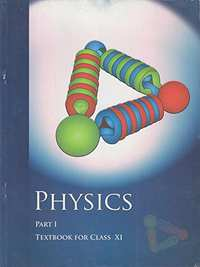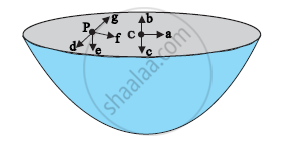CBSE (Science) Class 11CBSE
Share

NCERT solutions for Class 11 Physics chapter 8 - Gravitation

Physics Textbook for Class 11

NCERT Physics Class 11Chapter 8: Gravitation

Chapter 8: Gravitation solutions [Pages 201 - 202]

Q 1.1 | Page 201

You can shield a charge from electrical forces by putting it inside a hollow conductor. Can you shield a body from the gravitational influence of nearby matter by putting it inside a hollow sphere or by some other means?

Q 1.2 | Page 201

An astronaut inside a small space ship orbiting around the earth cannot detect gravity. If the space station orbiting around the earth has a large size, can he hope to detect gravity?

Q 1.3 | Page 201

If you compare the gravitational force on the Earth due to the Sun to that due to the Moon, you would find that the Sun’s pull is greater than the Moon’s pull. (You can check this yourself using the data available in the succeeding exercises). However, the tidal effect of the Moon’s pull is greater than the tidal effect of Sun. Why?

Q 2.1 | Page 201

Choose the correct alternative:

Acceleration due to gravity increases/decreases with increasing altitude.

Q 2.2 | Page 201

Choose the correct alternative:

Acceleration due to gravity increases/decreases with increasing depth. (assume the earth to be a sphere of uniform density).

Q 2.3 | Page 201

Choose the correct alternative:

Acceleration due to gravity is independent of mass of the earth/mass of the body.

Q 2.4 | Page 201

Choose the correct alternative:

The formula –G Mm(1/r2– 1/r1) is more/less accurate than the formula mg(r2– r1) for the difference of potential energy between two points r2and r1distance away from the centre of the earth.

Q 3 | Page 201

Suppose there existed a planet that went around the sun twice as fast as the earth.What would be its orbital size as compared to that of the earth?

Q 4 | Page 201

Io, one of the satellites of Jupiter, has an orbital period of 1.769 days and the radius of the orbit is 4.22 × 108 m. Show that the mass of Jupiter is about one-thousandth that of the sun.

Q 5 | Page 201

Let us assume that our galaxy consists of 2.5 × 1011 stars each of one solar mass. How long will a star at a distance of 50,000 ly from the galactic centre take to complete one revolution? Take the diameter of the Milky Way to be 105 ly

Q 6.1 | Page 201

Choose the correct alternative:

If the zero of potential energy is at infinity, the total energy of an orbiting satellite is negative of its kinetic/potential energy.

Q 6.2 | Page 201

Choose the correct alternative:

The energy required to launch an orbiting satellite out of earth’s gravitational influence is more/less than the energy required to project a stationary object at the same height (as the satellite) out of earth’s influence.

Q 7 | Page 201

Does the escape speed of a body from the earth depend on

(a) the mass of the body,

(b) the location from where it is projected,

(c) the direction of projection,

(d) the height of the location from where the body is launched?

Q 8 | Page 201

A comet orbits the Sun in a highly elliptical orbit. Does the comet have a constant (a) linear speed, (b) angular speed, (c) angular momentum, (d) kinetic energy, (e) potential energy, (f) total energy throughout its orbit? Neglect any mass loss of the comet when it comes very close to the Sun.

Q 9 | Page 201

Which of the following symptoms is likely to afflict an astronaut in space (a) swollen feet, (b) swollen face, (c) headache, (d) orientational problem?

Q 10 | Page 201

The gravitational intensity at the centre of a hemispherical shell of uniform mass density has the direction indicated by the arrow (see Fig 8.12) (i) a, (ii) b, (iii) c, (iv) 0.Q 11 | Page 202

Choose the correct answer from among the given ones:

For the problem 8.10, the direction of the gravitational intensity at an arbitrary point P is indicated by the arrow (i) d, (ii) e, (iii) f, (iv) g.

Q 12 | Page 202

A rocket is fired from the earth towards the sun. At what distance from the earth’s centre is the gravitational force on the rocket zero? Mass of the sun = 2 ×1030 kg, mass of the earth = 6 × 1024 kg. Neglect the effect of other planets etc. (orbital radius = 1.5 × 1011 m).

Q 13 | Page 202

How will you ‘weigh the sun’, that is estimate its mass? The mean orbital radius of the earth around the sun is 1.5 × 108 km.

Q 14 | Page 202

A Saturn year is 29.5 times the earth year. How far is the Saturn from the sun if the earth is 1.50 ×108 km away from the sun?

Q 15 | Page 202

A body weighs 63 N on the surface of the earth. What is the gravitational force on it due to the earth at a height equal to half the radius of the earth?

Q 16 | Page 202

Assuming the earth to be a sphere of uniform mass density, how much would a body weigh half way down to the centre of the earth if it weighed 250 N on the surface?

Q 17 | Page 202

A rocket is fired vertically with a speed of 5 km s–1 from the earth’s surface. How far from the earth does the rocket go before returning to the earth? Mass of the earth = 6.0 × 1024 kg; mean radius of the earth = 6.4 × 106 m; G= 6.67 × 10–11 N m2 kg2

Q 18 | Page 202

The escape speed of a projectile on the earth’s surface is 11.2 km s–1. A body is projected out with thrice this speed. What is the speed of the body far away from the earth? Ignore the presence of the sun and other planets

Q 19 | Page 202

A satellite orbits the earth at a height of 400 km above the surface. How much energy must be expended to rocket the satellite out of the earth’s gravitational influence? Mass of the satellite = 200 kg; mass of the earth = 6.0 ×1024 kg; radius of the earth = 6.4 ×106 m; G = 6.67 × 10–11 N m2 kg–2

Q 20 | Page 202

Two stars each of one solar mass (= 2× 1030 kg) are approaching each other for a head on collision. When they are a distance 109 km, their speeds are negligible. What is the speed with which they collide? The radius of each star is 104 km. Assume the stars to remain undistorted until they collide. (Use the known value of G).

Q 21 | Page 202

Two heavy spheres each of mass 100 kg and radius 0.10 m are placed 1.0 m apart on a horizontal table. What is the gravitational force and potential at the mid point of the line joining the centers of the spheres? Is an object placed at that point in equilibrium? If so, is the equilibrium stable or unstable?

Q 22 | Page 202

As you have learnt in the text, a geostationary satellite orbits the earth at a height of nearly 36,000 km from the surface of the earth. What is the potential due to earth’s gravity at the site of this satellite? (Take the potential energy at infinity to be zero). Mass of the earth = 6.0 × 1024 kg, radius = 6400 km

Q 23 | Page 202

A star 2.5 times the mass of the sun and collapsed to a size of 12 km rotates with a speed of 1.2 rev. per second. (Extremely compact stars of this kind are known as neutron stars. Certain stellar objects called pulsars belong to this category). Will an object placed on its equator remain stuck to its surface due to gravity? (mass of the sun = 2 x 1030 kg).

Q 24 | Page 202

A spaceship is stationed on Mars. How much energy must be expended on the spaceship to launch it out of the solar system? Mass of the space ship = 1000 kg; mass of the Sun = 2 × 1030 kg; mass of mars = 6.4 × 1023 kg; radius of mars = 3395 km; radius of the orbit of mars = 2.28 × 108kg; G= 6.67 × 10–11 m2kg–2.

Q 25 | Page 202

A rocket is fired ‘vertically’ from the surface of mars with a speed of 2 km s–1. If 20% of its initial energy is lost due to Martian atmospheric resistance, how far will the rocket go from the surface of mars before returning to it? Mass of mars = 6.4× 1023 kg; radius of mars = 3395 km; G = 6.67× 10-11 N m2 kg–2.

Chapter 8: Gravitation

NCERT Physics Class 11NCERT solutions for Class 11 Physics chapter 8 - Gravitation

NCERT solutions for Class 11 Physics chapter 8 (Gravitation) include all questions with solution and detail explanation. This will clear students doubts about any question and improve application skills while preparing for board exams. The detailed, step-by-step solutions will help you understand the concepts better and clear your confusions, if any. Shaalaa.com has the CBSE Physics Textbook for Class 11 solutions in a manner that help students grasp basic concepts better and faster.

Further, we at Shaalaa.com are providing such solutions so that students can prepare for written exams. NCERT textbook solutions can be a core help for self-study and acts as a perfect self-help guidance for students.

Concepts covered in Class 11 Physics chapter 8 Gravitation are Kepler’S Laws, Universal Law of Gravitation, The Gravitational Constant, Acceleration Due to Gravity of the Earth, Acceleration Due to Gravity Below and Above the Surface of Earth, Acceleration Due to Gravity and Its Variation with Altitude and Depth, Gravitational Potential Energy, Escape Speed, Earth Satellites, Energy of an Orbiting Satellite, Geostationary and Polar Satellites, Weightlessness, Escape Velocity, Orbital Velocity of a Satellite.

Using NCERT Class 11 solutions Gravitation exercise by students are an easy way to prepare for the exams, as they involve solutions arranged chapter-wise also page wise. The questions involved in NCERT Solutions are important questions that can be asked in the final exam. Maximum students of CBSE Class 11 prefer NCERT Textbook Solutions to score more in exam.

Get the free view of chapter 8 Gravitation Class 11 extra questions for Physics and can use Shaalaa.com to keep it handy for your exam preparation

S